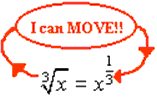# Rational Exponents

Definition of a Rational Exponent.

a m/n = ( na ) m

In general, if $a^{1/n} = \sqrt[n]{a}$ is a real number, then

a 1/ n = $a^{1/n} = \sqrt[n]{a}$

The denominator of a fractional exponent is equal to the index of the radical.

So 8 1/3 is the exponential form of the cube root of 8 , and $\sqrt{8}$ is its radical form .

Next, we ask: what sense can we make of a symbol like a 2/3 ? According to the rules of exponents:

a 2/3 = ( a 1/3 ) 2 or a 2/3 = ( a 2 ) 1/3

Example :

Use can use either of these rules to simplify the expression 8 2/3 as shown below.

Solution:

8 2/3 = (8 1/3 ) 2 = 2 2 = 4

or

8 2/3 = (8 2 ) 1/3 = 64 1/3 = 4

Notice that we get the same answer either way. However, to evaluate a fractional power, it is more efficient to take the root first.

 When you see a radical expression, you can convert it to a fractional power.The denominator of a fractional exponent indicates the root.

Specifically, $\sqrt{a} = a^{1/3}$ = a 1/3 or in general: $a^{m/n} = (\sqrt[n]{a})^m$ = a m / n

Notice: The index of the radical becomes the denominator of the rational power, and the exponent of the radicand (expression inside the radical) becomes the numerator.

27 -2/3 =
36 1/2 =